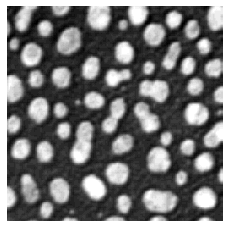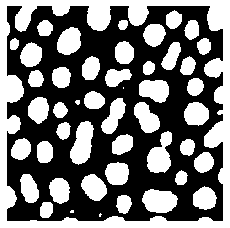# Indexing numpy arrays#

Indexing is the term used for selecting entries in an array, e.g. depending on its content. Again this is an operation that we cannot perform in a simple way using standard lists and where Numpy is very useful.

As a first simple example, we create here a 1D Numpy array:

import numpy
measurements = numpy.asarray([1, 17, 25, 3, 5, 26, 12])
measurements

array([ 1, 17, 25,  3,  5, 26, 12])


Our goal is now to recover in this array only values larger than a certain threshold, 10 for example. When we use simple Python variables, such comparisons can be done like this:

a = 5
b = a > 10
b

False


The output is a boolean value which takes the value True or False. Luckily we can do the same thing with Numpy arrays:

mask = measurements > 10

array([False,  True,  True, False, False,  True,  True])


Instead of getting a single boolean value we now get a Numpy array of booleans. We can now apply use this array as a mask to our data to retrieve a new array that only contains masked values (True in the mask array). For this we use again brackets (like for selecting rows and columns), but use the mask instead of indices:

measurements[mask]

array([17, 25, 26, 12])


## With images#

Instead of using this simle 1D array, we can perform the same operation on an entire image. Let’s import the blobs picture again:

from skimage.io import imread, imshow
from microfilm.microplot import microshow

image = imread("../../data/blobs.tif")

microshow(image);mask = image > 100

array([[False, False, False, ...,  True,  True,  True],
[False, False, False, ...,  True,  True,  True],
[False, False, False, ...,  True,  True,  True],
...,
[False, False, False, ..., False, False, False],
[False, False, False, ..., False, False, False],
[False, False, False, ..., False, False, False]])


Now we obtain a 2D array filled with boolean values. We can even look at it (white values are True):

microshow(mask);And now we can do indexing to recover all pixel values above our threshold of 100:

image[mask]

array([112, 152, 184, ..., 152, 128, 112], dtype=uint8)

len(image[mask])

24969


We have 24969 pixels above the threshold.

## Exercises#

Create a new mask for all pixel values above 200.

Apply the mask to retrieve a new array with numbers above 200.

Compute the average of all pixels above 200.